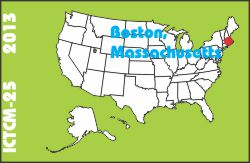#### Paper C032

 This is an electronic reprint, reproduced by permission of Pearson Education Inc. Originally appeared in the Proceedings of the Twenty-fifth Annual International Conference on Technology in Collegiate Mathematics, ISBN-10: 0133866726, Copyright (C) 2014 by Pearson Education, Inc.

# Is My Data Normal? Using Technology To Test For Normality

### James Graziose

Palm Beach State College

 Click to access this paper: paper.pdf

## ABSTRACT

In elementary statistics classes, many of our statistical tests that we perform on small data sets (n < 30) require the population from which the sample data was obtained be normally distributed. We explain to our students, a random variable X is normally distributed, or approximately normal, if the graph of the histogram is symmetric and bell-shaped or a normal-quantile plot which is linear. But in reality, we never obtain a perfect symmetric histogram or a normal quantile plot which is linear. Given a data set, which was obtained from a simple random sample whose distribution is unknown, we will apply two methods; a normalquantile plot and Lilliefors test to assess the normality of the random sample.

Keyword(s): statistics